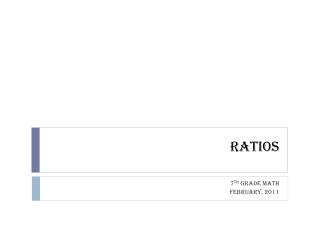DownloadDownload PresentationRatios

# Ratios

Télécharger la présentation## Ratios

- - - - - - - - - - - - - - - - - - - - - - - - - - - E N D - - - - - - - - - - - - - - - - - - - - - - - - - - -
##### Presentation Transcript

1. Ratios 7th Grade Math February, 2011

2. Ratio • A ___________ is a comparison of two numbers by division. • A ratio must be expressed in __________ . • Example #1: There are approximately 600 students at Lewisburg School. There are 30 teachers. What is the student to teacher ratio at Lewisburg School? Simplest Form 600/30 or 20/1

3. 3 ways to write a ratio! • There were 6 chocolate donuts in the box of 12 donuts. • Part to Whole = = ** This can be expressed as: ** 1:2 1 to 2 ALL THREE OF THESE MEAN ~ 1 CHOCOLATE DONUT FOR EVERY 2 DONUTS.

4. Equivalent Ratios • Two ratios are the same when put in simplest form. • Example – Are these two ratios equivalent?: • \$4 for every 16 ounces \$4/16 oz = • \$10 for every 40 ounces \$10/40 oz = • These two ratios are equivalent!! 1/4 1/4

5. Choose a partner and take turns taking each others pulse for 2 minutes. • Count the number of beats for each of you. • Write the ratio beats to minutes as a fraction. 1st block

6. A ___________ is a ratio that compares two different kinds of units. • Example: • Heartbeat example • Two Units = heartbeats and minutes Rate

7. Unit Rate • A ____________ is a ratio that is simplified so that it has a denominator of ONE unit. • Examples: • I made \$300 for 6 hours of work. Write the unit rate. • We drove 220 miles on 8 gallons of gas. Write the unit rate 300/6 = 50/1 220/8 = 27.5/1

8. How to calculate unit rate? • Easiest way is to divide BOTH the numerator AND the denominator by the denominator. • Example #1:

9. How to calculate unit rate? • Easiest way is to divide BOTH the numerator AND the denominator by the denominator. • Example #2:

10. Common Unit Rates

11. Common Unit Rates

12. Different Types of Ratios

13. Different Types of Ratios

14. Different Types of Ratios

15. Different Types of Ratios

16. Grocery Store Comparison – UNIT RATE! • Four Products: • Find the best price!!

17. Let’s try together: http://math.rice.edu/~lanius/proportions/rate.html

18. Practice • Small Group: • Number your paper from 1-12 • Deck of cards • LEVEL 0: INDIVIDUAL • Deal out cards evenly to each player • Answer that problem on your paper • Rotate the card (clockwise) to your partner • Continue until you’ve answered all cards • LEVEL 1: PARTNERS • Check your answers with your partners

19. Practice • Practice Problems: • Glencoe – Rates and Ratios • Rotate Problems through groups (5-10 minutes) each